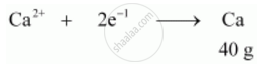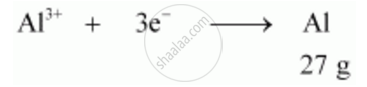# How Much Electricity in Terms of Faraday is Required to Produce - Chemistry

How much electricity in terms of Faraday is required to produce :

(a) 20 g of Ca from molten CaCl2

(b) 40 g of Al from molten Al203

(Given : Molar mass of Calcium and Aluminium are 40 g.mol-1 and 27 g.mol-1 respectively.)

#### Solution

a)Electricity required to produce 40g of calcium = 2F

Therefore, electricity required to produce 20 g of calcium

=(2xx20)/40F

=  1 F

b)Electricity required to produce 27g of Al = 3 F

Therefore, electricity required to produce 40 g of Al

=(3xx40)/27F

= 4.44 F

Concept: Electrolytic Cells and Electrolysis - Introduction
Is there an error in this question or solution?